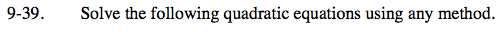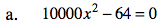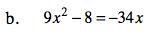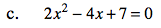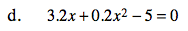### Home > CCA > Chapter 9 > Lesson 9.1.4 > Problem9-39

9-39.
1. Solve the following quadratic equations using any method. Homework Help ✎

1. 10000x2 − 64 = 0

2. 9x2 − 8 = −34x

3. 2x2 − 4x + 7 = 0

4. 3.2x + 0.2x2 − 5 = 0